Select Page

# Computer Architecture Today

Motivation

Number systems and computer arithmetics are essential for designing efficient hardware and software architecture. In particular, real-valued computation constitutes a crucial component in almost all forms of today’s computing systems from mobile devices to servers. IEEE 754 is a prominent standard established in 1985 for representing real-valued numbers in a floating-point format. Despite all its benefits, this number system suffers from a number of weaknesses.

• Large size for small numbers. The IEEE 754 standard defines two specific formats for single- and double-precision value representation using 32 and 64 bits, respectively. Numerical computation within a limited range of values in these formats may be largely inefficient. For example, computing dot-products of values within [-1, 1] requires only a tiny fraction of all possible numbers represented in either format.
• Limited precision. IEEE 754 has predefined fixed-size partitions for an exponent and a fraction. This may lead to a rounding error when representing real numbers; therefore, some floating-point numbers are not precise.
• Exceptional bit representations. IEEE 754 reserves several bits to represent NaNs, denormals, positive/negative zero and infinity. In addition to wasting some of the possible bit patterns, considering all the reserved patterns for computation adds further complexity to the floating-point processors.
• Breaking algebraic rules. The floating-point formats may break algebraic rules during computation. For instance, a floating-point addition is not always associative. The expression (x+y)+z results in 1, where the floating-point values are x = 1e30, y = -1e30 and z = 1 is 1. Using the same values, x+(y+z) results in 0.
• Producing inconsistent results. Consider two vectors Q = (3.2e7, 1, –1, 8.0e7) and W = (4.0e7, 1, –1, –1.6e7). The dot product Q.W is equal to 0 in single-precision (i.e., the float type in C); while, the right answer is 2. Using the floating-point representation, 80 intermediate bits are necessary to produce the correct answer in the double-precision format.
• Complex design and verification. Designing an efficient floating-point unit could be time-consuming due to the necessary components for handling rounding, exception, NaNs, denormals, mantissa alignment, etc. Moreover, verifying the floating-point design is a significant task because of dealing with numerous corner cases.

To overcome these challenges, various number systems and data representation techniques have been proposed to enhance or replace the floating-point numbers. A few examples of such techniques are Interval arithmeticuniversal number systems TypeI, and TypeII. The most recent floating-point number system invented by John L. Gustafson in 2017 is called posit that addresses many of the above-mentioned problems.

Posit Format

A generic posit format consists of a mandatory sign, one or multiple regime bits, multiple optional exponent bits, and multiple optional fraction bits (Fig. 1). The sign bit is 0 for positive numbers and 1 for negative ones. The number of regime bits is dynamic following a special encoding. After the sign bit, the regime includes a run of 0 or 1, which is terminated by an opposite bit () or at the end of the number format. Similarly, the number of bits for the exponent and fraction is dynamic. A posit number includes the exponent and fraction only if necessary.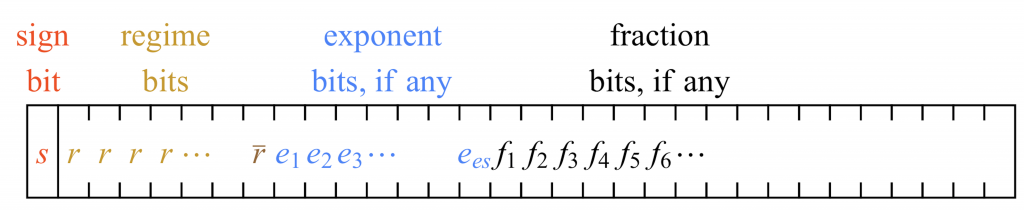Fig.1: General posit format for finite, nonzero values-color codes.

To understand how the regime bits represent numbers, consider the binary numbers in Fig. 2.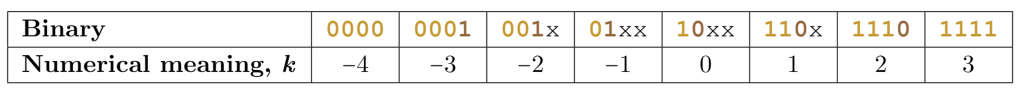Fig. 2: Decimal values of regime bits (“x”  means don’t care).

Let m be the number of identical bits in the regime bits (amber color). If the first bit is zero, the number of zeros (m) represents a negative value (-m). Otherwise, the number of ones minus one (m-1) represents a positive value (m-1). The regime bits realize a scale factor of useedk, where useed = 22es.

Exponent e (blue bits) is regarded as an unsigned integer to realize another scale 2e. Unlike IEEE 754, posit does not use bias for the exponent. Each exponent may be up to a predefined number of bits (es). The remaining bits after the regime and the exponent are used for the fraction (f). Similar to IEEE 754, the fraction includes a hidden bit, which is always 1 as posit does not have any denormal number. Overall, an n-bit posit number (p) can represent the following numbers.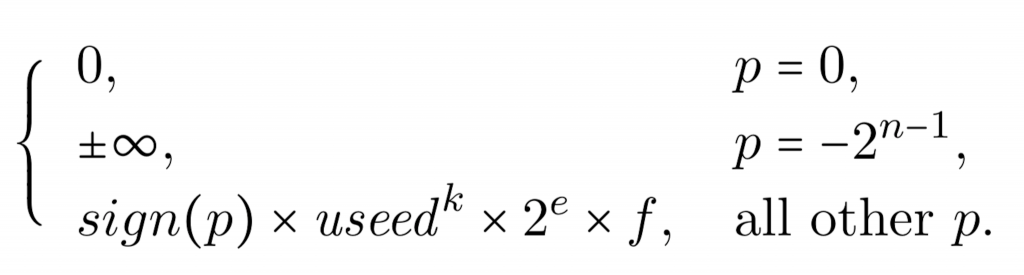For instance, Fig.3 represents 477/134217728 ≈ 3.55393 × 10-6 with es=3.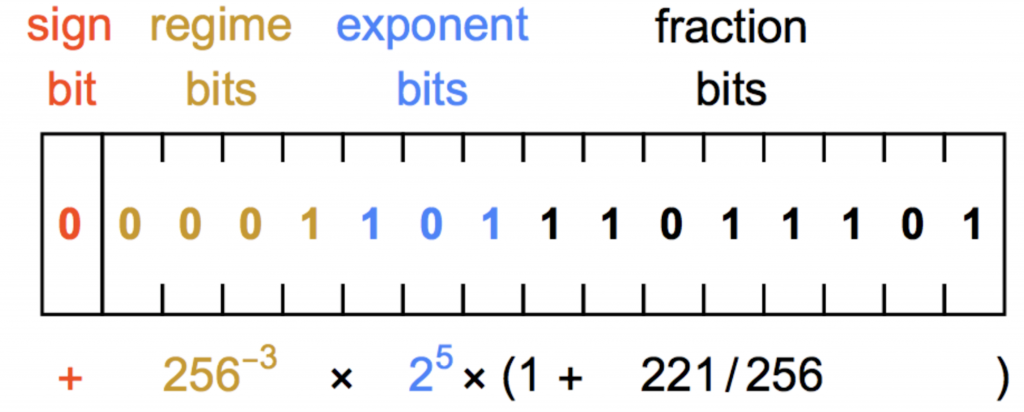Fig. 3: Example of a posit number and its decimal value

Posit Number Construction

Fig. 4 shows values for a 3-bit posit format with n = 3 and es = 1. There are only two reserve representations: 0 (all 0 bits) and ±∞ (1 followed by all 0 bits). A total of 8 values may be represented using 3 bits.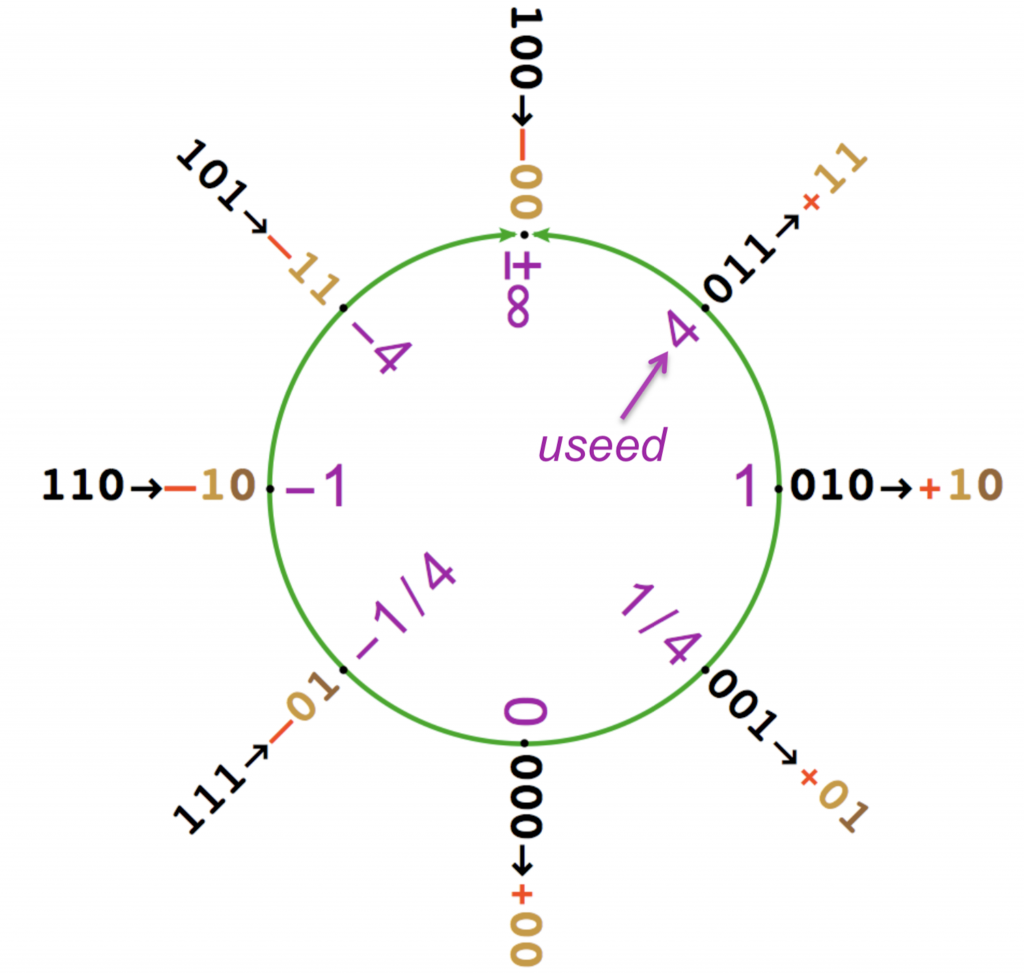Fig. 4: Values for a 3-bit posit

Similar to the floating-point numbers, appending 0 to a number does not change its value, whereas appending a 1 results in a new value between two existing numbers on the ring (Fig. 5, and 6).  The new posit value may be (1) between the maxvalue (useed) and ±∞, the new value is maxvalue × useed, (2) between the existing values x = 2m and y = 2n , where |m-n|> 1, the new value is xy (add a new exponent bit), or (3) between the existing x and y values next to it, which is (x + y)/2( add a new fraction bit).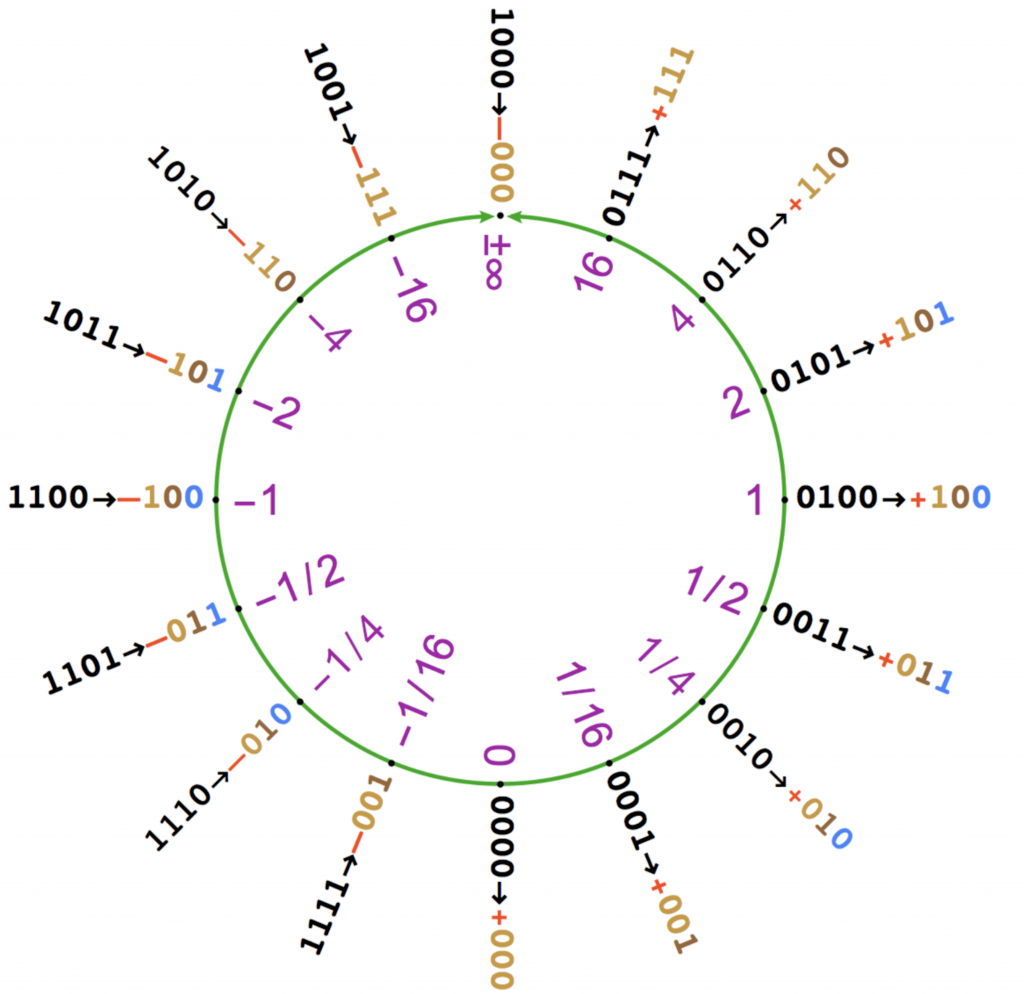Fig. 5: Values for a 4-bit posit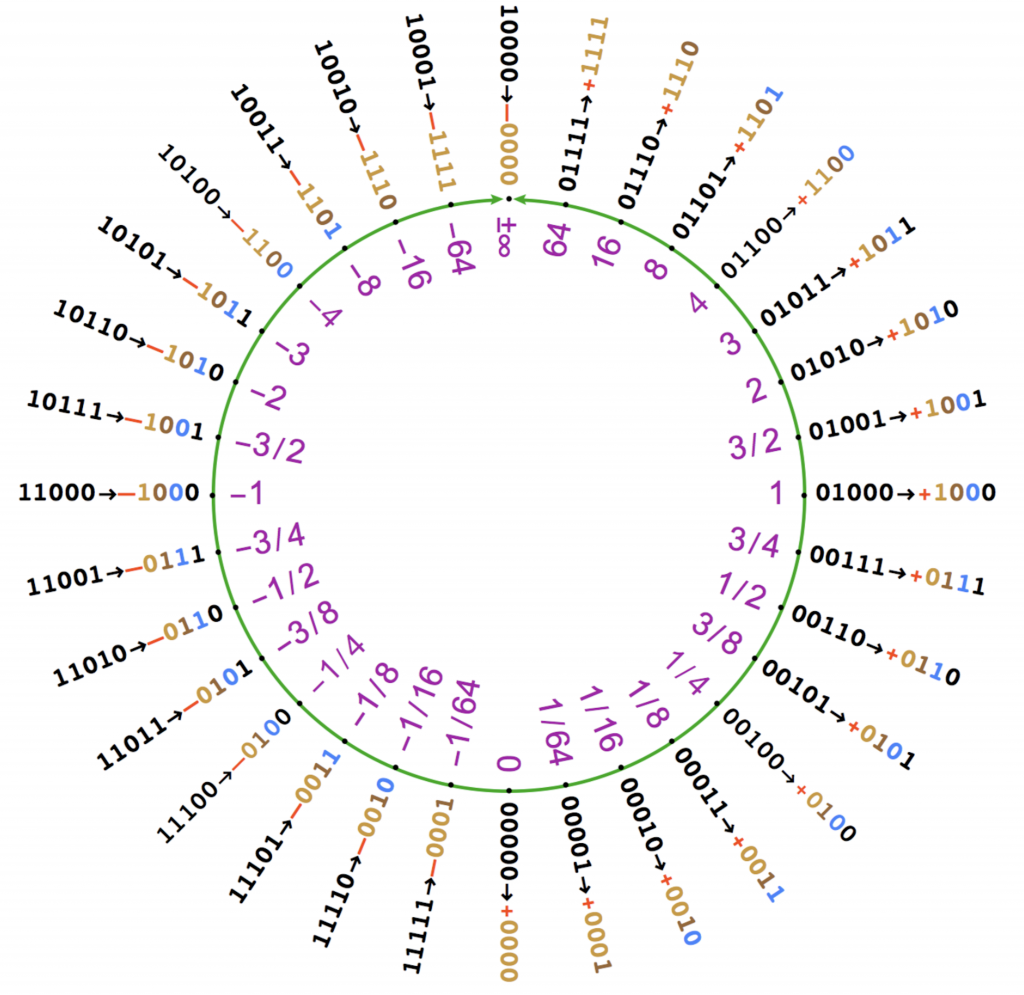Fig. 6: Values of a 5-bit posit

Posit vs. IEEE 754 standard

Unique Value Representation. In the posit format, f(a) is always equal to f(b) if a and b are equal, where f is a function. In the IEEE 754, the reciprocals of positive and negative zeros are +∞, −∞, respectively. Moreover, the negative zero equals positive zero. This implies +∞ = -∞ which is not true. In a floating-point comparison (a == b), the result is always false if either a or b is NaN. This even holds if a and b has the same bit representation. In posits, however, a and b are equal if they use the same bit patterns; otherwise, they are not equal. Moreover, the result of an arithmetic operation would be the same over different hardware systems. For instance, in the case of the Q.W example at the beginning, posit needs only 24 bits to generate the correct result.

No Gradual Underflow. IEEE 754 faces an underflow problem when the exact result of an operation is nonzero but smaller than the smallest normalized number. This problem is alleviated by rounding values. However, this may result in a denormal number. As a result, some fraction digits are transferred to the exponent for representing smaller numbers. This is known as gradual underflow. Handling gradual underflow is complicated and is supported in software by some IEEE compliant microprocessors. The posit number system does not encounter this problem due to supporting a tapered precision, where numbers with small exponents are represented more accurately than the numbers with large magnitude exponents.

Holding Algebraic Rules Across Formats. Unlike IEEE 754, posit holds the associativity of addition. Moreover, computing values across multiple posit formats with different sizes is guaranteed to produce the same value.

Exception Handling. While there are 14 representations of Nans in IEEE 754, there is no “NaN” in posits. Moreover, posit has single representations for 0 and ∞. Overall, it makes the computation with the posit numbers simpler than IEEE 754. In the case of exceptions (e.g., division by zero), the interrupt handler is expected to report the error and its cause to the application.

Inefficiencies of Posit Numbers. Despite all the expected benefits of posit numbers, the floating-point format has one important advantage over posit, which is the fixed bit format for the exponent and fraction. As a result, parallel decoding may be used for extracting the exponent and fraction of floating-point numbers. Whereas, decoding the posit fields may only be done serially due to using dynamic ranges. However, not having NaNs and denormals simplifies the circuit to some extent. Nevertheless, unlike the floating-point system, posit is a newly proposed number system yet to be investigated thoroughly. To the best of authors’ knowledge, there is no fabricated chip using posit arithmetic to date.

Number systems can significantly influence the energy-efficiency of computation and data movement in computer systems. For instance, recent studies show how a posit number system may improve the performance of neural networks. As the need for energy-efficient computing is never-ending, it becomes increasingly important to design an efficient number system for future computing systems.

About the authors: Payman Behnam is a graduate researcher at the Energy-Efficient Computer Architecture Laboratory, University of Utah, where he is involved in designing novel memory systems and energy-efficient accelerators for computer vision and machine learning applications. Mahdi Nazm Bojnordi is an assistant professor of the School of Computing at the University of Utah, Salt Lake City, UT.

Disclaimer: These posts are written by individual contributors to share their thoughts on the Computer Architecture Today blog for the benefit of the community. Any views or opinions represented in this blog are personal, belong solely to the blog author and do not represent those of ACM SIGARCH or its parent organization, ACM.